Next: Interpretation Up: N finite slits: combining Previous: N finite slits: combining

## Analysis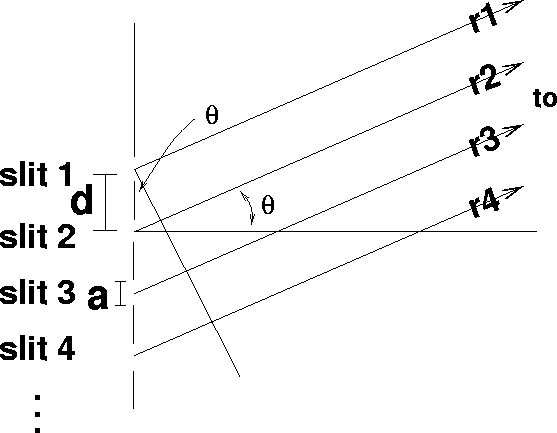Figure 10: N finite-slit interference experiment

Finally, we consider the pattern created by N identical slits at spacing d, but each with finite width a (Figure 10). As you might expect, this case becomes a simple combination of the result for the finite slit with the result for N slits.

The reasoning for this is as follows. If you view any one of the N finite slits, say slit n, by itself from different angles, it will appear to change its intensity according to the single-slit formula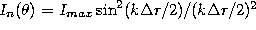, where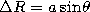. If we now open all N slits at once and view the result at angle, what we see is the result of the interference of N slits, each with this same intensity. Thus, if we set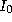from Eq. 17 equal to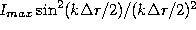, we will find the result for N finite slits. This result is just the product of our two previous results: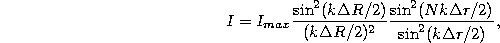where,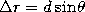, and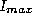is the central maximum intensity from any one of the slits alone.

Tomas Arias
Thu Sep 13 15:26:14 EDT 2001